Open AccessArticle

# Global Climate Data Processing and Mapping of Degradation Mechanisms and Degradation Rates of PV Modules

byJulián Ascencio-Vásquez 1,*,Ismail Kaaya 2,3,Kristijan Brecl 1,Karl-Anders Weiss 2 andMarko Topič 11
Faculty of Electrical Engineering, University of Ljubljana, Tržaška cesta 25, 1000 Ljubljana, Slovenia
2
Fraunhofer Institute of Solar Energy, Heidenhofstr. 2, 79110 Freiburg im Breisgau, Germany
3
School of Industrial Engineering, University of Malaga, 29016 Malaga, Andalucia, Spain
*
Author to whom correspondence should be addressed.
Energies 2019, 12(24), 4749; https://doi.org/10.3390/en12244749
Received: 17 October 2019 / Revised: 4 December 2019 / Accepted: 7 December 2019 / Published: 12 December 2019

Keywords:

## 1. Introduction

The current worldwide energy transition from conventional to renewable energy sources already proves and even more forecasts the expansion of photovoltaic systems to new and diverse locations. On a global scale, we are rapidly approaching the so-called “Terawatt-scale Photovoltaics” era, which indicates the surpass of 1 TW of installed capacity of photovoltaic (PV) systems and highlights an important milestone for the PV industry . This milestone is also boosted by the decrease in prices of the PV technologies, which are already the cheapest source of electricity in many countries of Europe .
Studies at a global scale present the mapping of PV performance indicators (e.g., performance ratio and energy yield) using different Geographic Information Systems (GIS) datasets and approaches [12,13,14]. Regarding the long-term operation of PV systems, usually this issue is simplified by considering a typical value (i.e., 0.5%/a for crystalline PV modules), also stated on manufacturer performance warranties. However, it is known that the impact of climate on material properties and energy production will vary over time and location. Interesting approaches to calculate the degradation rates have been proposed in references [10,15]. Both models consider as main climate degradation factors the average temperatures, temperature changes, humidity, and ultraviolet (UV) irradiation, which is in line with indoor testing and standards for PV reliability. However, those models have not been applied at global scale and a PV degradation mapping has not been published so far.
In this paper, the ERA5 climate reanalysis dataset  is processed to extract and further to model the essential climate variables for the study of PV degradation (temperature, humidity, and UV irradiation). The estimated climate data is compared and validated with real ground-measurements taken from different sources [17,18,19]. Then, the module temperature and degradation rates are estimated globally. Using the Köppen-Geiger-Photovoltaic (KGPV) climate classification , the spatial distribution of degradation rates in view of climate zones is evaluated. Then, the temporal evolution of degradation rates compared with the global increase in ambient temperature is presented. The global mapping of degradation mechanisms and total degradation rates are provided, together with a categorization of degradation rates in Europe and worldwide.

## 2. Climate Data Processing

The studies over large geographical regions can be made by processing GIS data estimated from Numerical Weather Predictions (NWP) including satellite or reanalysis models . Even though satellite-based estimations can be more accurate than the reanalysis-based ones, the advantage of the second is the possibility to extract all the essential variables together in the same dataset, without gaps and identical timestamps.
One of the latest datasets released is the “ERA5” created by the European Centre for Medium-Range Weather Forecasts (ECMWF). In comparison with previous datasets (e.g., ERA-Interim), ERA5 provides a spatial resolution of 31 km and temporal resolution of hourly data from 1979 . Also in the literature, the improvement on the accuracy of the global horizontal irradiance ($G H I$) is reported .
Although not all variables required are directly available from the ERA5, we calculate the missing local climate variables, wind speed ($W S$), relative humidity ($R H$), and UV irradiance ($U V$), using existing models.

#### 2.1. Local Climate Variables

While high wind speed ($W S$) can increase mechanical loads on the PV installation  and be a trigger of further degradation processes, we use it only to estimate the PV module temperature ($T m o d$) due to the related cooling effect on materials. The $W S$ is calculated and height-corrected according to Equations (1) and (2) [22,23], where $u ¯ w i n d$ and $v ¯ w i n d$ are the vector components of the wind, $h E R A$ is the height from ground which the wind is modelled in the ERA5 dataset, and $h m o d$ is the assumed height of the PV modules equal to 2 m. The 2 m height assumed is in accordance with the height of modelled ambient temperature ($T a m b$) and dew point temperature ($T d e w$) given by the ECMWF.
$W S = ( h m o d h E R A ) 0.2 · W S E R A$
The relative humidity ($R H$) is also not extracted directly from ERA5, so it is estimated using Equations (3) and (4). The saturation water vapor pressure ($W V P$) over water and ice is calculated using Buck’s Formula [24,25] from the dew point temperature ($T D e w$) and ambient temperature ($T a m b$).
UV irradiance given in the ERA5 dataset covers a wavelength range up to 440 nm. However, this variable is usually referred for wavelengths below 400 nm. This distinction can cause large differences of the estimated UV irradiation. For example, measurements in Ljubljana, Slovenia lead to an overestimation of 45% of the energy in the UV part (see Figure 1).
For this reason, we neglect the $UV$ irradiance data given by ERA5, and model it up to 400 nm using a method proposed in ref.  and expressed in Equations (5)–(8), which is based on the clearness index ($k t$) and the global horizontal irradiance ($G H I$). The $k t$ is calculated by dividing the $G H I$ and the top-of-atmosphere irradiance extracted from ERA5. Unfortunately, the lack of valid measurements disallows that we validate the model worldwide.
$k t ∗ = m a x ( 0.1 , m i n ( k t , 0.7 ) )$
$U V = U V A + U V B$
The GIS data extracted and computed is compared with 15 ground meteorological stations involved in the World Radiation Monitoring Center - Baseline Surface Radiation Network (WRMC-BSRN) , one station in Alice Springs, Australia provided by the Desert Knowledge Australia (DKA) Solar Centre  and one station in the Atacama Desert provided by Universidad de Chile . The station locations are shown in Figure 2 and more details are presented in Table A1 in the Appendix A. At these stations, $G H I$, $T a m b$, and $R H$ are measured. The comparison is carried out on a daily average resolution for the years 2016, 2017, and 2018, except for the Chilean station where the time frame ranges from 2010 to 2014. We use the inverse distance weighting (IDW) as interpolation method  to calculate the estimated values at specific location reducing the geographical mismatch between measured and computed values.
The time-series for each location are compared by the cumulative distribution function (CDF) and the coefficient-of-determination (R2). In Figure 3, locations with high and low accuracy are presented, while the rest of the locations are presented in Table A2 in the Appendix A. For example, on one hand, data in Cabauw (The Netherlands) show almost perfect fitting for the three variables ($T a m b$, $G H I$, and $R H$), evidencing that ERA5 can be used as synthetic data in some locations. On the other hand, in Howrah (India), the accuracy of $G H I$ and $R H$ is low but could be improved with a post-processing algorithm, e.g., ref. .
An excellent agreement of $T a m b$ was noticed possibly because of the high number of observational data in ERA5. $G H I$ and $R H$ also show good performance even though they are purely modelled.

#### 2.2. Operating Conditions of PV Modules

One of the most important climatic loads to analyze the degradation is the PV module temperature ($T m o d$). Typically this variable is estimated by using the Ross model , which is a function of the ambient temperature, irradiance and the Ross coefficient ($k R o s s$) as presented in Equation (9). However, a higher accuracy of estimations under different climate conditions for crystalline silicon PV modules can be achieved by using the Faiman model (see Equation (10)) [22,30]. In Figure 4, a visual comparison of the estimated $T m o d$ is illustrated. The main differences are presented in areas with high wind speed where the cooling effect will be taken into account when using the Faiman model.
Further calculations in this paper are based on the Faiman model to estimate the PV module temperature as a function of the , $G H I$, and , considering $u 0$ equal to 26.9 W/m2/°C and $u 1$ equal to 6.2 Ws/m3/°C , which are typical values reported for c-Si PV modules in the open-rack mounting configuration.
$T m o d = T a m b + k R o s s · G H I$
$T m o d = T a m b + G H I u 0 + u 1 · W S$

## 3. Spatial Distribution of PV Degradation Rates

Degradation mechanisms will be triggered not only from one individual degradation factor but due to a combination of them. In ref. , three degradation processes are defined and empirically expressed due to the combination of climate degradation factors: Hydrolysis-degradation ($k H )$ related to the effect of temperature and humidity, photo-degradation ($k P$) depends on temperature, humidity and UV irradiance, and thermo-mechanical-degradation ($k T m$) due to high temperature and temperature differences. The models are presented in Equations (11) to (14):
$k H = A H · r h e f f n · exp ( − E H k B · T m ) ,$
$k P = A P · ( U V d o s e ) X · ( 1 + r h e f f n ) · exp ( − E P k B · T m ) ,$
$k T m = A T m · ( Δ T ) θ · C N · exp ( − E T m k B · T U ) ,$
$k T = A N ∏ i = 1 n ( 1 + k i ) − 1 ,$
where the parameters are:
 $k H$: Hydrolysis degradation rate (%) $r h e f f$: effective relative humidity $k P$: Photo-degradation rate (%) $U V d o s e$: integral UV dose (kW/m²) $k T m$: Thermomechanical degradation rate (%) $T m$: average module temperature $k T$: Total degradation rate (%) $T U$: upper module temperature $k B$: Boltzmann constant (8.62 × 10−5) $T L$: lower module temperature $E H$: Activation Energy for Hydrolysis degradation (eV) $Δ T = T U − T L$: temperature difference $E P$: Activation Energy for Photo-degradation (eV) $n$: model parameter that indicates the impact of RH on power degradation. $E T m$: Activation Energy for Thermomechanical degradation (eV) $X$: model parameter that indicates the impact of UV dose on power degradation. $A H$: Pre-exponential constant for Hydrolysis degradation $θ$: model parameter that indicates the impact of $Δ T$ on power degradation. $A P$: Pre-exponential constant for Photo-degradation $C N$: Cycling rate $A T m$: Pre-exponential constant for Thermomechanical degradation $A N$: normalization constant of the physical quantities (a−2/%)
The degradation mechanisms are quantified by using the fitting coefficients published in ref.  for a high-performance monocrystalline silicon PV module installed in the open-rack mounting configuration.
Further on, the calculated degradation rates can be directly related to climate zones. To simplify the spatial distribution analysis of degradation rates, we use the Köppen-Geiger-Photovoltaic (KGPV) scheme proposed in ref.  to compare the climate zones in terms of annual degradation rates, as shown in Figure 5 and Figure 6. Each KGPV climate zone is defined by two letters, the first one implies the relation of temperature and precipitation (TP-zones) and the second is related to the irradiation level, as H-zones. The definition of each letter is as follows:
 Temperature-Precipitation (TP) Zones Irradiation (H) Zones $A$: Tropical climate $K$: Very high irradiation $B$: Desert climate $H$: High irradiation $C$: Steppe climate $M$: Medium irradiation $E$: Temperate climate $L$: Low irradiation $D$: Cold climate $F$: Polar climate
Hydrolysis-degradation presents the smallest contribution in almost all the KGPV zones, but is considerably higher for the tropical climates (AH and AK), which zones are related to high precipitation levels (humid areas) and temperature levels. This process can provoke moisture ingress leading to delamination of polymers or corrosion of solder bonds .
Photo-degradation has the second-highest contribution to the total degradation rate. This indicator combines the humidity, temperature, and UV irradiance impacting the PV module. The impact is similar to hydrolysis-degradation but higher in terms of absolute values due to the process triggered by UV irradiation. For desert areas, even though the UV irradiation is high, the low humidity in the air decreases the estimated damage of the PV cells due to this mechanism. The photo-degradation is considerable high in tropical zones (AH and AK) due to the high climatic stresses of all variables (temperature, humidity, and UV irradiation).
Thermo-mechanical degradation exhibits the highest contribution to the total degradation rate in all zones, except in the AH zone, where the temperature variations are minimal. This parameter is affected by seasonal temperature cycling (the difference between the maximum and minimum temperature of the year) and also the annual average maximum ambient temperature.
The total degradation rates calculated by the combination of the previous three degradation mechanisms Equation (13) are also evaluated per each KGPV climate zone. In accordance with the literature , the highest degradation rate is identified in tropical areas (hot and humid). Interestingly, the AK (tropical with very high irradiation) presents lower degradation than the AH (tropical with high irradiation), due to lower photo-degradation contribution (related to lower humidity).
The steppe climate has higher humidity than the deserts and therefore, the total degradation rate is increased by higher hydrolysis-degradation and photo-degradation. As expected CK (steppe with very high irradiation) might be more stressful for PV modules than CH (steppe with high irradiation), due to higher UV irradiation.
Temperate climates (DM and DH) result in average degradation rates of 0.42%/a and 0.58%/a, respectively. Those climate zones are predominant in Europe, and their values are in accordance with the typical 0.5%/a degradation rate assumed along within the PV community. However, the spread of the values can range around ± 0.3%/a in the temperature zones across Europe.
Cold and polar areas present average degradation rates around or below 0.2%/a. In real operating conditions, we expect higher values due to external degradation factors, such as snow accumulation over the PV systems or mechanical loads due to wind gusts which are not included in the calculations as yet.
Desert areas exhibit similar average degradation rates than temperate areas. In absolute climate values, desert areas are hotter and dryer than temperate areas. However, in the relative contribution of degradation mechanisms, the high thermomechanical stress together with low hydrolysis and photo-degradation makes the average similar to the moderate climate stressors of the temperate climate. In real operating conditions, external degradation factors, such as soiling might increase the degradation rate if taken into account, but the degradation presented here assumes only gradual and non-reversible degradation processes.

The worldwide mapping of the degradation mechanisms (hydrolysis-degradation, photo-degradation and thermomechanical-degradation) are presented in Figure A1 in the Appendix B. As mentioned before, the simulations are based on the degradation model developed in ref. , and coefficients fitted for a specific PV module (high-performance mono-crystalline silicon PV module). The climate datasets used were extracted, modelled and averaged from the ERA5 reanalysis dataset for the years 2016, 2017, and 2018.
Figure A2 in the Appendix B shows the calculated worldwide degradation rate combining the three degradation mechanisms based on the main climate degradation factors.
To facilitate the visualization and possible use of our degradation maps, we categorize the locations into bins of 0.2%/a ranging from 0% to 0.8%/a for Europe and ranging from 0% to 1.4%/a around the World. The categorized maps are shown in Figure 7 and Figure A3, respectively. The total degradation rates could reach 0.8%/a in the hottest areas of the south of Spain and Portugal for Europe, and globally the highest degradation rates (above 1.4%/a) are identified in locations next to the equator line.

## 5. Uncertainty over Time-Temporal Evolution

The temporal evolution of the climate also might be a factor to consider while estimating the degradation rates of PV modules. In ref.  the decrease of the Performance Ratio due to climate change effect is already reported. Hereby, the annual degradation rates are simulated for the PV module described previously, using historical data from ERA5. For convenience in terms of computational resources, degradation rates based on hourly data are calculated only for the years 1980, 1990, 2000, 2010, 2016, 2017, and 2018. The ambient temperature is extracted for every year from 1979 to 2018.
In Figure 8, the evolution of the ambient temperature and degradation rates is shown. The global calculations represent the average values for land-surface between the Latitudes −60° and 60°. We notice that an increase in the ambient temperature also increases the degradation of the PV modules over time.

## 6. Discussions

The used degradation model currently takes into account pure climate degradation factors and it does not include temporal or external degradation factors or failure modes such as light-induced degradation (LID), light and elevated temperature degradation (LeTID), potential-induced-degradation (PID) or mechanical damage due to wind or snow loads. Neither the underperformance due to soiling or snow covering is considered. Also, the final results of total degradation rates represent the value at steady conditions (for example, after LID). The calculations presented in this paper are related to a specific monocrystalline silicon PV module, and the values could be different for devices with different BOM but we expect the trend to remain the same for all crystalline silicon technologies.
The correlation with the KGPV climate zones shows a good agreement with the literature. Tropical climates are again presented as the harshest for PV modules due to the high humidity and high temperature. Desert and steppe climates present high degradation rates due to high daily temperature changes, even excluding soiling. Under no consideration of wind and snow loads, the temperate, cold and polar climates present low degradation rates.
Even though the results are coherent, some climate and degradation modelling topics need further investigation: (1) lack of UV irradiation measurements spread around the world does not allow a representative validation of models, (2) moisture ingress and related triggering of degradation processes, such as corrosion or delamination, need to be understood for different interactions of materials, and (3) the understanding of degradation mechanisms under high UV irradiance and extremely low humidity exposure.
Despite the current limitations, the average degradation rates calculated agree with the typical values used in manufacturer warranties and developers, showing that in the worst case (e.g., tropical climates) an average degradation of 1%/a can offer more than 20 years of performance above 80%, and the global average degradation rate of 0.5%/a could promise a lifetime operation of 40 years. Additionally, the mapping and results presented can give a new perspective of the climatic stresses worldwide when considering the installation of PV modules at different locations. Also, the high resolution of the GIS data, including ambient temperature, UV irradiation and humidity, together with the module operating temperature, helps to identify relevant variables for PV systems where measured data is not available. The degradation mechanisms and the total degradation rates calculated for the land-surface are available as Supplementary Materials attached to this article.

## 7. Conclusions

To validate the estimation of climate variables, we extracted the time series of 17 ground measurement stations from the World Radiation Monitoring Center - Baseline Surface Radiation Network (WRMC-BSRN), Desert Knowledge Australia (DKA) Solar Centre, and Universidad de Chile which include ambient temperature, global horizontal irradiance and relative humidity. The cumulative distribution function and coefficient-of-determination (R2) were calculated to prove the good agreement between the ground measurements and the modelled data from ERA5 interpolated using the Inverse-Distance-Weighting method. For the validation, we considered daily average values from 2016 to 2018.
In terms of global spatial distribution, we found a clear correlation between the Köppen-Geiger-Photovoltaic (KGPV) climate classification and the estimated degradation rates. In the temperate zones of Europe, the average degradation rate is in accordance with the typical degradation rate of 0.5%/a considered widely by the PV community, however, this value can vary around ± 0.3%/a for a specific year.
From our calculations, thermomechanical degradation is the harshest for the studied PV module in nearly all climate zones, presenting the highest impact in very high irradiation zones, such as CK, BK, and AK. Photo-degradation and hydrolysis-degradation show similar global spatial distribution, but the former is higher since it also comprises UV irradiation as a degradation factor.
The temporal evolution of the degradation rates is directly correlated with the global ambient temperature and it is evidence that climate change could impact the long-term performance of PV systems.
We developed new maps for degradation mechanisms and the total degradation rate for the studied PV module which can be directly integrated as a new layer over the KGPV climate classification map and provide a rapid understanding of performance and degradation globally. However, due to the high uncertainty in the real degradation rate of PV systems (solar resource, methodology of calculation, quality of operational data, bill-of-materials of PV modules, etc.), the maps are presented as a guide to identify possible risk areas in terms of climate stress but not to give quantified degradation rates for specific locations for any PV module or PV system.

## Supplementary Materials

The datasets generated in this article are available online as Mendeley data at https://data.mendeley.com/datasets/3nt652dwwx/1.

## Author Contributions

J.A.-V., I.K. worked on the data processing, simulations and analyses. K.B., K.-A.W., and M.T. discussed the results and helped to improve the schemes. All the authors discussed the results and contributed, read and commented on the manuscript.

## Funding

This project received funding from the European Union’s H2020 programme SOLAR-TRAIN under grant agreement No 721452 and research programme P2-0197 funded by the Slovenia Research Agency.

## Acknowledgments

The authors would like to thank the organizations ECMWF, DKA Solar Centre, Universidad de Chile and WRMC-BSRN for providing climate datasets.

## Conflicts of Interest

The authors declare no conflict of interest.

## Appendix A. Meteorological Stations and Data Validation

Table A1. Details of meteorological stations used for the validation of $G H I$, $T a m b$, and $R H$.
Table A1. Details of meteorological stations used for the validation of $G H I$, $T a m b$, and $R H$.
LabelLocationLatitudeLongitudeElevation [m]Available Time Frame
ALIAlice Springs, Australia−23.76133.885602008-09 to 2019-11
CABCabauw, Netherlands52.04.902005-02 to 2019-05
CARCarpentras, France44.15.11001996-09 to 2018-12
CNRPamplona, Spain42.8−1.64712009-07 to 2019-02
E13Oklahoma, USA36.6−97.53181994-01 to 2017-05
FUAFukuoka, Japan33.6130.432010-04 to 2019-04
GOBGobabeb, Namibia−23.615.04072012-05 to 2019-05
GURGurgaon, India28.477.22592014-07 to 2019-01
HOWHowrah, India22.688.3512014-10 to 2019-01
LINLindenberg, Germany52.214.11251994-10 to 2017-01
LRCVirginia, USA37.1−76.432014-12 to 2019-05
PALPalaiseau, France48.72.21562003-06 to 2019-02
PAYPayerne, Switzerland46.86.94911992-10 to 2019-03
SAPSapporo, Japan43.1141.3172010-04 to 2019-04
SPASan Pedro de Atacama, Chile−22.98−68.1623902009-05 to 2014-06
TATTateno, Japan36.1140.1251996-02 to 2019-03
TIRTiruvallur, India13.180.0362014-08 to 2019-01
Table A2. Cumulative Distribution Function (CDF) for $G H I$, $T a m b$ and to compare ground stations from BSRN, DKA Solar Centre and Universidad de Chile with modelled and interpolated data from ERA5 climate reanalysis.
Table A2. Cumulative Distribution Function (CDF) for $G H I$, $T a m b$ and to compare ground stations from BSRN, DKA Solar Centre and Universidad de Chile with modelled and interpolated data from ERA5 climate reanalysis.
Cumulative Distribution FunctionsVar.R2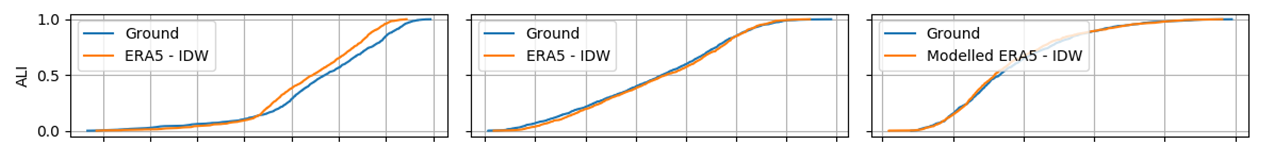$G H I T a m b R H$0.76
0.98
0.91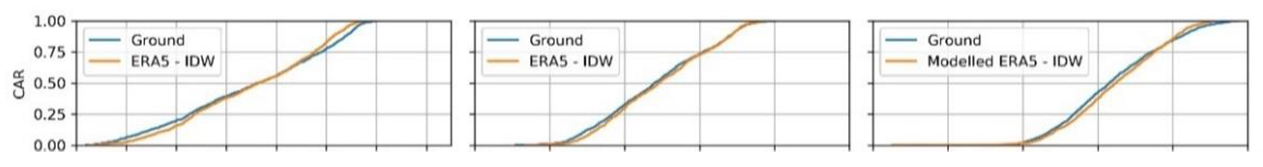$G H I T a m b R H$0.89
0.98
0.82$G H I T a m b R H$0.78
0.98
0.64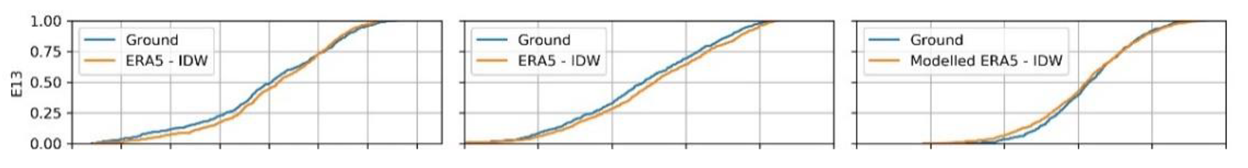$G H I T a m b R H$0.81
0.99
0.85$G H I T a m b R H$0.82
0.99
0.80$G H I T a m b R H$0.65
0.72
0.71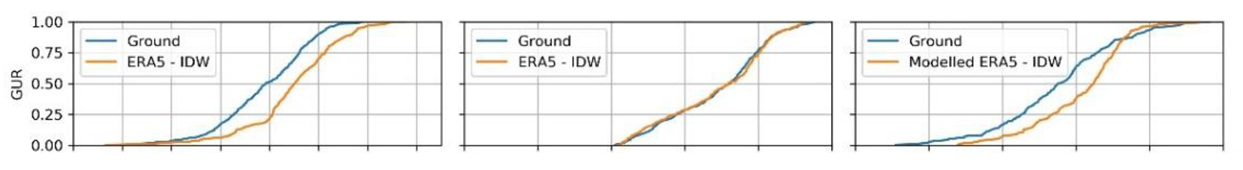$G H I T a m b R H$0.63
0.98
0.82$G H I T a m b R H$0.90
0.99
0.93$G H I T a m b R H$0.89
0.99
0.78$G H I T a m b R H$0.87
0.99
0.83$G H I T a m b R H$0.79
0.98
0.47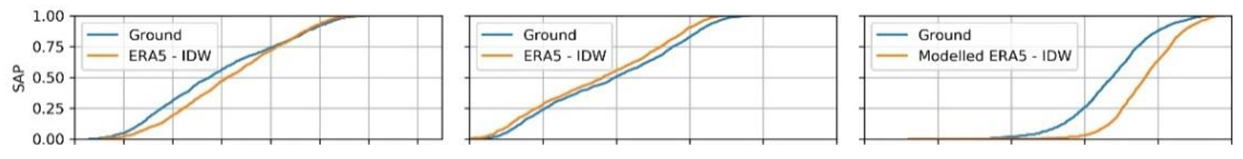$G H I T a m b R H$0.72
0.99
0.62$G H I T a m b R H$0.79
0.84
0.75$G H I T a m b R H$0.86
0.99
0.86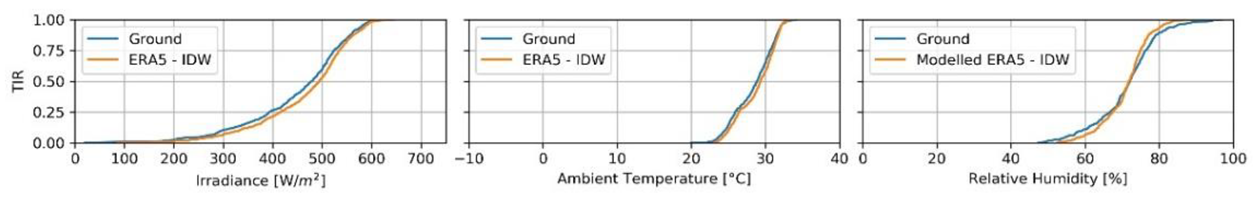$G H I T a m b R H$0.49
0.96
0.75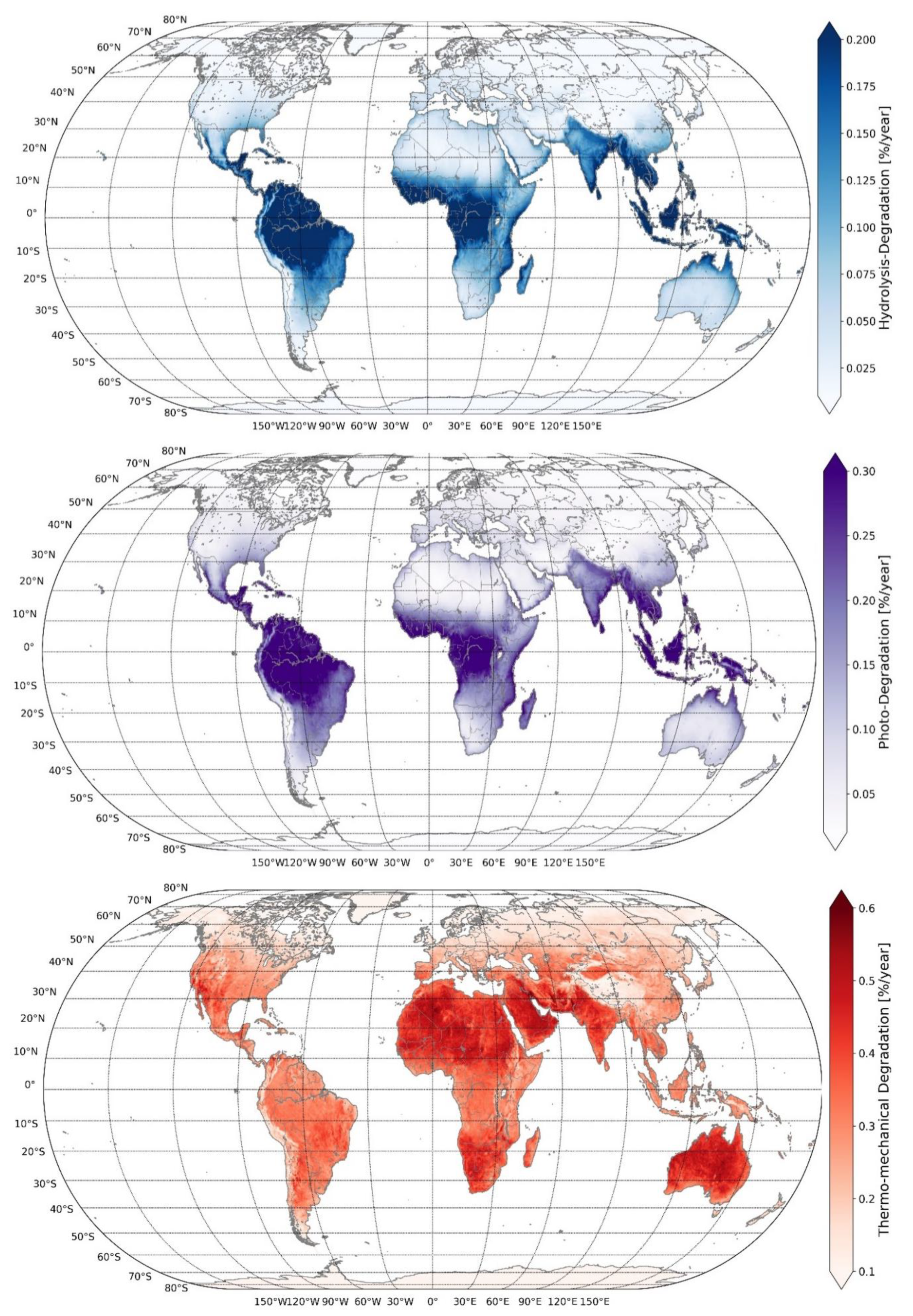Figure A2. Total degradation rates for a specific mono-crystalline silicon PV module using the Kaaya model. Climate data from ERA5 for the average between 2016 and 2018.
Figure A2. Total degradation rates for a specific mono-crystalline silicon PV module using the Kaaya model. Climate data from ERA5 for the average between 2016 and 2018.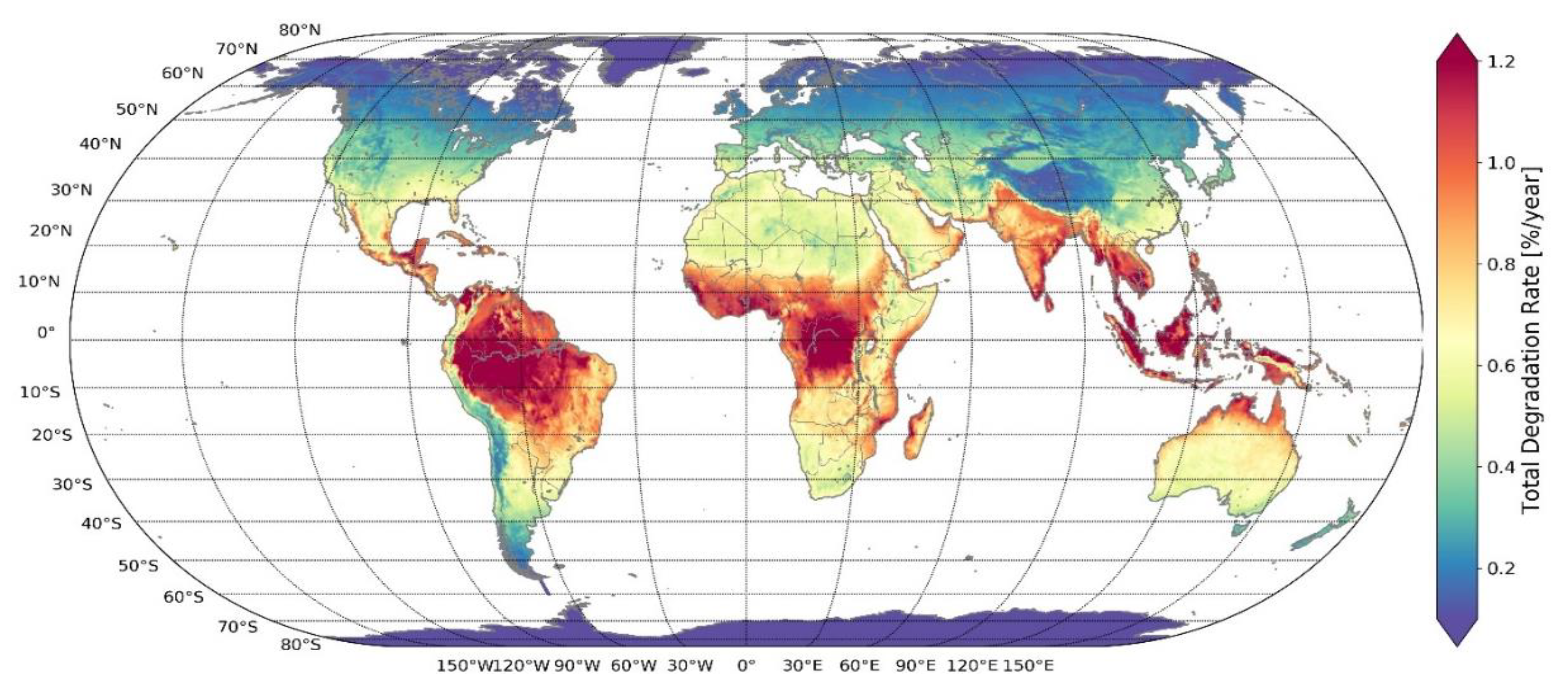Figure A3. Categorization of total degradation rates for a specific mono-crystalline silicon PV module using the Kaaya model. Climate data from ERA5 for the average between 2016 and 2018.
Figure A3. Categorization of total degradation rates for a specific mono-crystalline silicon PV module using the Kaaya model. Climate data from ERA5 for the average between 2016 and 2018.## References

1. Haegel, N.M.; Atwater, H.; Barnes, T.; Breyer, C.; Burrell, A.; Chiang, Y.-M.; De Wolf, S.; Dimmler, B.; Feldman, D.; Glunz, S.; et al. Terawatt-scale photovoltaics: Transform global energy. Science 2019, 364, 836–838. [Google Scholar] [CrossRef] [PubMed]
2. Vartiainen, E.; Masson, G.; Breyer, C.; Moser, D.; Román Medina, E. Impact of weighted average cost of capital, capital expenditure, and other parameters on future utility-scale PV levelised cost of electricity. Prog. Photovolt. Res. Appl. 2019. [Google Scholar] [CrossRef]
3. Jordan, D.C.; Wohlgemuth, J.H.; Kurtz, S.R. Technology and Climate Trends in PV Module Degradation. In Proceedings of the 27th European Photovoltaic Solar Energy Conference and Exhibition, Frankfurt, Germany, 24–28 September 2012. [Google Scholar]
4. Jordan, D.C.; Kurtz, S.R.; VanSant, K.; Newmiller, J. Compendium of photovoltaic degradation rates: Photovoltaic degradation rates. Prog. Photovolt. Res. Appl. 2016, 24, 978–989. [Google Scholar] [CrossRef]
5. Jordan, D.C.; Silverman, T.J.; Wohlgemuth, J.H.; Kurtz, S.R.; VanSant, K.T. Photovoltaic failure and degradation modes: PV failure and degradation modes. Prog. Photovolt. Res. Appl. 2017, 25, 318–326. [Google Scholar] [CrossRef]
6. Omazic, A.; Oreski, G.; Halwachs, M.; Eder, G.C.; Hirschl, C.; Neumaier, L.; Pinter, G.; Erceg, M. Relation between degradation of polymeric components in crystalline silicon PV module and climatic conditions: A literature review. Sol. Energy Mater. Sol. Cells 2019, 192, 123–133. [Google Scholar] [CrossRef]
7. Halwachs, M.; Neumaier, L.; Vollert, N.; Maul, L.; Dimitriadis, S.; Voronko, Y.; Eder, G.C.; Omazic, A.; Mühleisen, W.; Hirschl, C.; et al. Statistical evaluation of PV system performance and failure data among different climate zones. Renew. Energy 2019, 139, 1040–1060. [Google Scholar] [CrossRef]
8. Eder, G.C.; Voronko, Y.; Dimitriadis, S.; Knöbl, K.; Újvári, G.; Berger, K.A.; Halwachs, M.; Neumaier, L.; Hirschl, C. Climate specific accelerated ageing tests and evaluation of ageing induced electrical, physical, and chemical changes. Prog. Photovolt. Res. Appl. 2018, 1–16. [Google Scholar] [CrossRef]
9. Dubey, R.; Chattopadhyay, S.; Kuthanazhi, V.; Kottantharayil, A.; Singh Solanki, C.; Arora, B.M.; Narasimhan, K.L.; Vasi, J.; Bora, B.; Singh, Y.K.; et al. Comprehensive study of performance degradation of field-mounted photovoltaic modules in India. Energy Sci. Eng. 2017, 5, 51–64. [Google Scholar] [CrossRef]
10. Kaaya, I.; Koehl, M.; Mehilli, A.P.; de Cardona Mariano, S.; Weiss, K.A. Modeling Outdoor Service Lifetime Prediction of PV Modules: Effects of Combined Climatic Stressors on PV Module Power Degradation. IEEE J. Photovolt. 2019, 9, 1105–1112. [Google Scholar] [CrossRef]
11. Lindig, S.; Kaaya, I.; Weis, K.-A.; Moser, D.; Topic, M. Review of Statistical and Analytical Degradation Models for Photovoltaic Modules and Systems as Well as Related Improvements. IEEE J. Photovolt. 2018, 8, 1773–1786. [Google Scholar] [CrossRef]
12. Ascencio-Vásquez, J.; Brecl, K.; Topič, M. Methodology of Köppen-Geiger-Photovoltaic climate classification and implications to worldwide mapping of PV system performance. Sol. Energy 2019, 191, 672–685. [Google Scholar] [CrossRef]
13. Peters, I.M.; Liu, H.; Reindl, T.; Buonassisi, T. Global Prediction of Photovoltaic Field Performance Differences Using Open-Source Satellite Data. Joule 2018, 2, 307–322. [Google Scholar] [CrossRef]
14. Victoria, M.; Andresen, G.B. Using validated reanalysis data to investigate the impact of the PV system configurations at high penetration levels in European countries. Prog. Photovolt. Res. Appl. 2019, 27, 576–592. [Google Scholar] [CrossRef]
15. Bala Subramaniyan, A.; Pan, R.; Kuitche, J.; TamizhMani, G. Quantification of Environmental Effects on PV Module Degradation: A Physics-Based Data-Driven Modeling Method. IEEE J. Photovolt. 2018, 8, 1289–1296. [Google Scholar] [CrossRef]
16. Copernicus Climate Change Service (C3S) ERA5: Fifth Generation of ECMWF Atmospheric Reanalyses of the Global Climate. Copernicus Climate Change Service Climate Data Store (CDS) 2017. Available online: https://cds.climate.copernicus.eu/cdsapp#!/home (accessed on 13 October 2019).
17. Driemel, A.; Augustine, J.; Behrens, K.; Colle, S.; Cox, C.; Cuevas-Agulló, E.; Denn, F.M.; Duprat, T.; Fukuda, M.; Grobe, H.; et al. Baseline Surface Radiation Network (BSRN): Structure and data description (1992–2017). Earth Syst. Sci. Data 2018, 10, 1491–1501. [Google Scholar] [CrossRef]
19. Molina, A.; Falvey, M.; Rondanelli, R. A solar radiation database for Chile. Sci. Rep. 2017, 7, 14823. [Google Scholar] [CrossRef]
20. Urraca, R.; Huld, T.; Martinez-de-Pison, F.J.; Sanz-Garcia, A. Sources of uncertainty in annual global horizontal irradiance data. Sol. Energy 2018, 170, 873–884. [Google Scholar] [CrossRef]
21. Camus, C.; Offermann, P.; Weissmann, M.; Buerhop, C.; Hauch, J.; Brabec, C.J. Site-specific assessment of mechanical loads on photovoltaic modules from meteorological reanalysis data. Sol. Energy 2019, 188, 1134–1145. [Google Scholar] [CrossRef]
22. Schwingshackl, C.; Petitta, M.; Wagner, J.E.; Belluardo, G.; Moser, D.; Castelli, M.; Zebisch, M.; Tetzlaff, A. Wind Effect on PV Module Temperature: Analysis of Different Techniques for an Accurate Estimation. Energy Procedia 2013, 40, 77–86. [Google Scholar] [CrossRef]
23. Huld, T.; Amillo, A. Estimating PV Module Performance over Large Geographical Regions: The Role of Irradiance, Air Temperature, Wind Speed and Solar Spectrum. Energies 2015, 8, 5159–5181. [Google Scholar] [CrossRef]
24. Buck, A.L. New Equations for Computing Vapor Pressure and Enhancement Factor. J. Appl. Meteorol. 1981, 20, 1527–1532. [Google Scholar] [CrossRef]
25. Buck Research Instruments, LLC. CR-1A User’s Manual; Buck Research Instruments, LLC: Aurora, CO, USA, 2012. [Google Scholar]
26. Wald, L. A Simple Algorithm for the Computation of the Spectral Distribution of the Solar Irradiance at Surface; Research Report; Mines ParisTech: Paris, France, 2018. [Google Scholar]
27. Kuo, P.-H.; Chen, H.-C.; Huang, C.-J. Solar Radiation Estimation Algorithm and Field Verification in Taiwan. Energies 2018, 11, 1374. [Google Scholar] [CrossRef]
28. Yang, D. Post-processing of NWP forecasts using ground or satellite-derived data through kernel conditional density estimation. J. Renew. Sustain. Energy 2019, 11, 026101. [Google Scholar] [CrossRef]
29. Skoplaki, E.; Palyvos, J.A. Operating temperature of photovoltaic modules: A survey of pertinent correlations. Renew. Energy 2009, 34, 23–29. [Google Scholar] [CrossRef]
30. Faiman, D. Assessing the outdoor operating temperature of photovoltaic modules. Prog. Photovolt. Res. Appl. 2008, 16, 307–315. [Google Scholar] [CrossRef]
31. Koehl, M.; Heck, M.; Wiesmeier, S. Categorization of weathering stresses for photovoltaic modules. Energy Sci. Eng. 2018, 6, 93–111. [Google Scholar] [CrossRef]
Figure 1. (a) Spectral irradiance from 280 nm to 440 nm under a day of cloudy sky conditions. (b) Spectral irradiance from 280 nm to 440 nm under a day of clear sky conditions.
Figure 1. (a) Spectral irradiance from 280 nm to 440 nm under a day of cloudy sky conditions. (b) Spectral irradiance from 280 nm to 440 nm under a day of clear sky conditions.Figure 2. Location of meteorological stations used to compare the $G H I$, $T a m b$, and $R H$ with the modelled data from ERA5 reanalysis dataset.
Figure 2. Location of meteorological stations used to compare the $G H I$, $T a m b$, and $R H$ with the modelled data from ERA5 reanalysis dataset.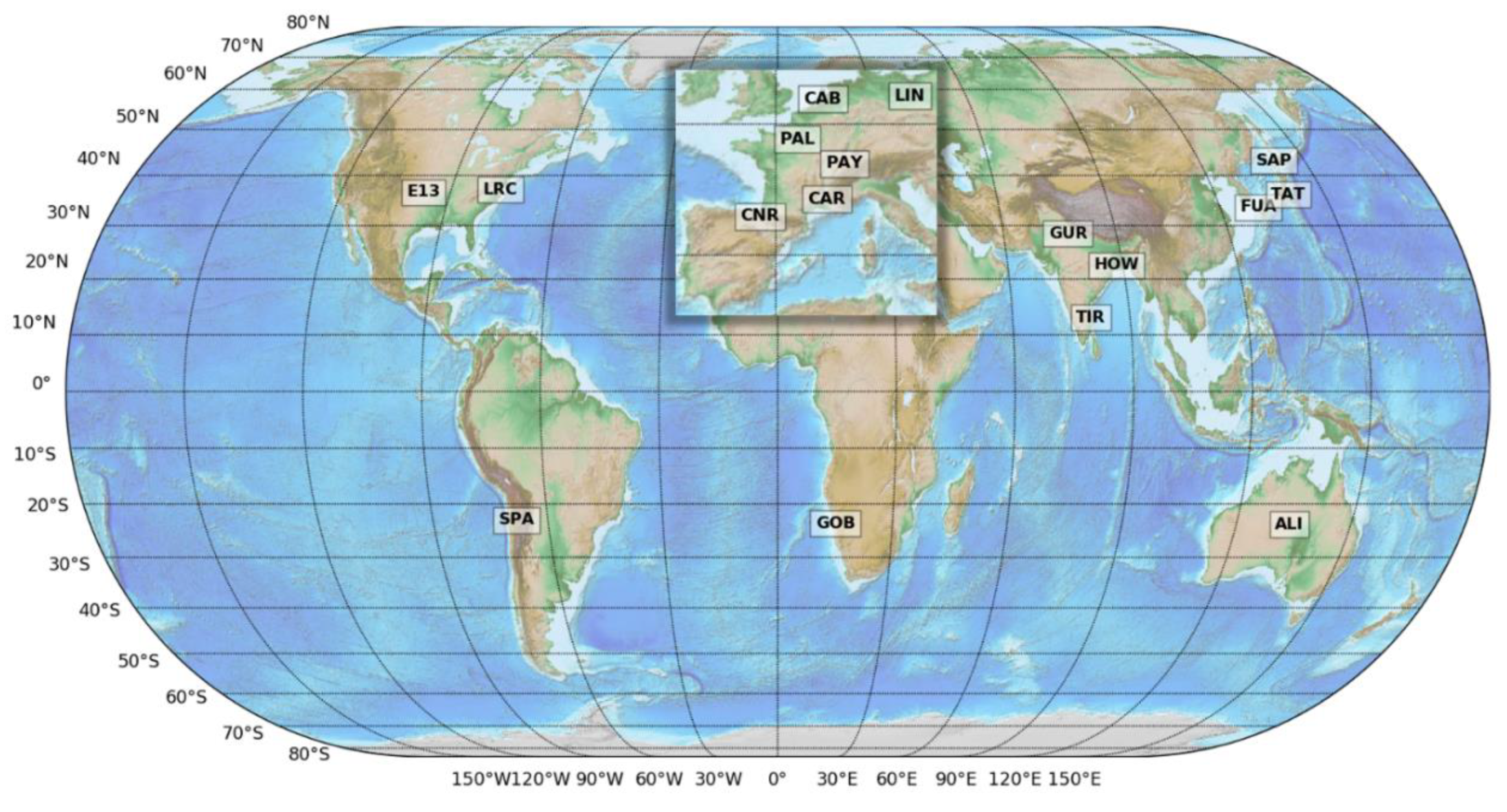Figure 3. Cumulative distribution functions (CDFs) and statistical indicators for comparison of ground-measurements and modelled data from the ERA5 reanalysis dataset and the inverse distance weighting (IDW) interpolation. We present a high accuracy and low accuracy case, Cabauw (CAB, Lopik, Utrecht, The Netherlands) and Howrah (HOW, West Bengal, India). The comparison of the other 15 locations is presented in Table A2 in the Appendix A.
Figure 3. Cumulative distribution functions (CDFs) and statistical indicators for comparison of ground-measurements and modelled data from the ERA5 reanalysis dataset and the inverse distance weighting (IDW) interpolation. We present a high accuracy and low accuracy case, Cabauw (CAB, Lopik, Utrecht, The Netherlands) and Howrah (HOW, West Bengal, India). The comparison of the other 15 locations is presented in Table A2 in the Appendix A.Figure 4. (a) Maximal module temperature using the Ross model. (b) Maximal module temperature using the Faiman model. (c) Absolute difference between the estimated $T m o d$ using Faiman and Ross models. Annual average data from 2016 to 2018.
Figure 4. (a) Maximal module temperature using the Ross model. (b) Maximal module temperature using the Faiman model. (c) Absolute difference between the estimated $T m o d$ using Faiman and Ross models. Annual average data from 2016 to 2018.Figure 5. Spatial distribution of the degradation mechanisms in view of the Köppen-Geiger-Photovoltaic (KGPV) climate zones.
Figure 5. Spatial distribution of the degradation mechanisms in view of the Köppen-Geiger-Photovoltaic (KGPV) climate zones.Figure 6. Spatial distribution of the total degradation rates in view of the KGPV climate zones. The average of total degradation rate per climate zone is indicated below each label in %/a.
Figure 6. Spatial distribution of the total degradation rates in view of the KGPV climate zones. The average of total degradation rate per climate zone is indicated below each label in %/a.Figure 7. European categorization of total degradation rate based on temperature, humidity, and UV irradiance for a specific monocrystalline silicon PV module.
Figure 7. European categorization of total degradation rate based on temperature, humidity, and UV irradiance for a specific monocrystalline silicon PV module.Figure 8. Temporal evolution of the global ambient temperature and the total degradation rates. The calculations are limited to the land-surface between the latitudes −60° and 60°.
Figure 8. Temporal evolution of the global ambient temperature and the total degradation rates. The calculations are limited to the land-surface between the latitudes −60° and 60°.